PPWIZARD is a free preprocessor for HTML, REXX, Visual Basic or any text files.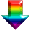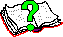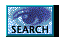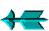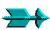\ -> Rexx -> Inbuilt PPWIZARD Functions -> ArraySort()

 ArraySort()

This is an inbuilt function function provided by PPWIZARD.

This function is used to sort a rexx array. The "array" has numeric indexes with the ".0" element holding the number of elements. The array can hold numbers or strings.

The sort is always ascending. You can use the ArrayReverse() routine if required.

The function takes 1 or more parameters as follows:

1. The name of the array (don't include the dot).

2. Separate Key - "FROM" value (see below).

If this value is "@" then the "FROM" value is set to lower case characters and the "TO" value is set to the upper case values (for a case insensitive sort).

To configure the case conversion see the "Upper/Lower Case Configuration" section.

3. Separate Key - "TO" value (see below).

4. This is an optional parameter. Do you want a strict compare? If you do then pass 'Y'. A strict compare does not ignore leading and trailing whitespace, however it won't correctly sort numbers.

The routine returns the number of items in the array.

If you are sorting a very large array with a fixed known name (or want multiple sort key, or sort multiple arrays) then you might wish to have a look at the faster and more powerful PPWSORT.H header file.

The sorting routines are implemented as rexx code to be portable, for large numbers of items you may find it a bit slow, if so have a look at "REGUTIL" from http://www.interlog.com/~ptjm/.

 SEPARATE SORT KEY

Unless a "FROM" value is supplied the sort key is the same as the data being sorted.

Sometimes you wish to sort data using a different key, an example of this is a case insensitive sort. PPWIZARD will create a separate sort array which is used for sorting the data if you request this.

There are basically 3 modes to how PPWIZARD builds the sort array:

1. Extract columns
If the "FROM" value is numeric (actually integer) then you wish specific columns to be extracted out of the data array.

The "FROM value is the starting column (first column is 1), while the "TO" value is the ending column (if not supplied the rest of the string is used).

2. Translate Data
The "FROM" value is a string containing one or more characters you wish to translate.

Each character gets translated to one at the same position in the "TO" string (for example if FROM="AB" and TO="ab", then "A" is translated to "a" and "B" to "b").

3. Use Supplied Sort Key Array
If the "TO" value is not supplied then the "FROM" value is actually the name of an array (don't include the trailing dot) in which you have prebuilt the sort keys you wish used. The elements of this sort key array must correspond element for element with the target array.

 Silly Example

```;--- Set up the array ------------------------
#DefineRexx ''
A.1 = "ASDFG";
A.2 = "4123";
A.3 = "61743";
A.4 = "1678";
A.0 = 4;
#DefineRexx

;--- Sort array & display --------------------
#evaluate '' ^call ArraySort "A"^
#evaluate '' ^do I = 1 to A.0; say '"'a.i'"'; end; say ''^

;--- Sort array (start column 3) & display ---
#evaluate '' ^call ArraySort "A", 3^
#evaluate '' ^do I = 1 to A.0; say '"'a.i'"'; end;

;--- Sort Array (case insensitive) ------------------------------------------
#DefineRexx ''
call ArraySort "A", xrange('a', 'z'), xrange('A', 'Z');  ;;One way
call ArraySort "A", '@'                                  ;;Another
#DefineRexx
```

Saturday May 28 2022 at 2:55pm